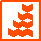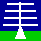HOME ANNOUNCEMENTS CONTACTS DATA INSTRUMENTS MAIN MENU MISCELLANEOUS PLOTS PUBLICATIONS SCIENCE WIND-PROFILERPRINCIPLES For this page: INTERNAL LINKS THE NERC MST RADAR FACILITY AT ABERYSTWYTH WIND-PROFILER BEAM FORMATION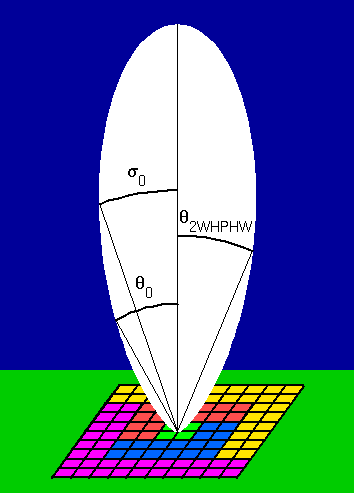The polar diagram of the main-lobe for a wind-profiler radar beam typically has a Gaussian form. The beam width is inversely proportional to both the frequency of operation and the horizontal dimensions of the antenna; for a width of the order of a few degrees, dimensions of the order of 100 m are required for lower-VHF radars and of the order of a few metres for UHF radars. The different conventions for describing the beam width are described below. In order to reduce the side-lobe levels, it is common to use an antenna array which allows the transmitted and received power to be tapered off away from the centre. This is illustrated for the case of the NERC MST Radar's antenna array which is composed of a 10 × 10 grid of 'quads' (each quad being composed of a 2 × 2 sub-grid of 4-element Yagi aerials); the division of the antenna array into quads has an additional significance in terms of beam steering. Equal power weighting is given to the central section of 4 quads (green), the two middle sections of 16 quads each (red and blue), and the two outer sections of 32 quads each (yellow and magenta). In the transmit mode, each of the five sections is connected to a separate 32 kW peak-power transmitter. It is common to see the (two-way) polar diagram of the main-lobe for a (vertically directed) radar beam described as: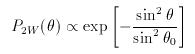where θ is the angle from zenith and θ0 is the e-1 half-width, i.e. the half-width of the beam at which P2W drops to 0.368 of its peak value; the term two-way refers to the fact that the same antenna is used for transmission and reception. The beam width can alternatively be described in terms of the (two-way) half-power half-width, θ2WHPHW, i.e. the half-width of the beam at which P2W drops to 0.5 of its peak value; the relationship between &theta0 and θ2WHPHW can be derived from the ratio of P2W(θ2WHPHW) to P2W(0) through the following steps: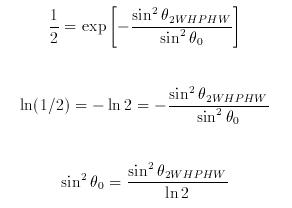However, the beam width is often described in terms of the ONE-WAY half-power half-width, θ1WHPHW, i.e. for the antenna used only for transmission OR reception. The one-way polar diagram of the main-lobe is simply the square root of the two-way case, i.e:and it can be shown, using the same steps as above for the two-way case, that &theta0 and θ1WHPHW are related through the expression: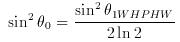Finally, it is useful to define the two-way polar diagram in terms of standard Gaussian notation: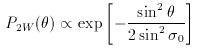i.e. with a factor of 2 in the denominator of the exponent so that the measure of beam width, σ0, is the standard deviation, i.e. the e-1/2 half-width or the half-width of the beam at which P2W drops to 0.607 of its peak value; &sigma0 and θ0 are related through the expression:so that: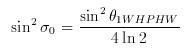This description of beam width is required for correcting (standard deviation) radar return spectral widths for the effects of beam broadening. Note, however, that the spectral widths in the existing files of NERC MST Radar data are defined in a non-standard way; the values must therefore be multiplied by a scaling factor of 1.25 (explained in the file format page). The NERC MST Radar has a one-way half-power half-width of 1.5°, i.e. sin2σ0 = 2.47 × 10-4. Boundary-layer wind-profilers tend to have one-way half-power half-widths of the order of 5°. Internal Links: Return to top of page Wind-profiler beam steering Correction of spectral widths for the effects of beam broadening File format for existing MST Radar radial dataPage maintained by David Hooper Last updated 17th December 2002